# Approximate controllability of some nonlinear systems in Banach spaces

## Abstract

In this paper, abstract results concerning the approximate controllability of semilinear evolution systems in a separable reflexive Banach space are obtained. An approximate controllability result for semilinear systems is obtained by means of Schauder’s fixed-point theorem under the compactness assumption of the linear operator involved. It is also proven that the controllability of the linear system implies the controllability of the associated semilinear system. Then the obtained results are applied to derive sufficient conditions for the approximate controllability of the semilinear fractional integrodifferential equations in Banach spaces and heat equations.

## 1 Introduction

The problems of controllability of infinite dimensional nonlinear (fractional) systems were studied widely by many authors; see  and the references therein. The approximate controllability of nonlinear systems when the semigroup $S\left(t\right)$, $t>0$, generated by A is compact has been studied by many authors. The results of Zhou  and Naito  give sufficient conditions on B with finite dimensional range or necessary and sufficient conditions based on more strict assumptions on B. Li and Yong in  studied the same problem assuming the approximate controllability of the associated linear system under arbitrary perturbation in ${L}_{\mathrm{\infty }}\left(I,L\left(X\right)\right)$. Bian  investigated the approximate controllability for a class of semilinear systems. For abstract nonlinear systems, Carmichael and Quinn  used the Banach fixed-point theorem to obtain a local exact controllability in the case of nonlinearities with small Lipschitz constants. Zhang  studied the local exact controllability of semilinear evolution systems. Naito  and Seidman  used Schauder’s fixed-point theorem to prove invariance of the reachable set under nonlinear perturbations. Other related abstract results were given by Lasiecka and Triggiani .

In recent years, controllability problems for various types of nonlinear fractional dynamical systems in infinite dimensional spaces have been considered in many publications. An extensive list of these publications focused on the complete and approximate controllability of the fractional dynamical systems can be found (see [15, 7, 947]). A pioneering work has been reported by Bashirov and Mahmudov , Dauer and Mahmudov  and Mahmudov . Sakthivel et al.  studied the approximate controllability of nonlinear deterministic and stochastic evolution systems with unbounded delay in abstract spaces. Klamka  derived a set of sufficient conditions for constrained local controllability near the origin for semilinear dynamical control systems. Wang and Zhou  investigated the complete controllability of fractional evolution systems without assuming the compactness of characteristic solution operators. Sukavanam and Kumar  obtained a new set of sufficient conditions for the approximate controllability of a class of semilinear delay control systems of fractional order by using the contraction principle and Schauder’s fixed-point theorem.

Consider an abstract semilinear equation

$y={y}_{0}+LBv+LF\left(y,v\right),$
(1)

and define the following sets: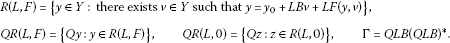Here Y, X are separable reflexive Banach spaces and V is a Hilbert space, $B\in L\left(V,Y\right)$, $L\in L\left(Y,Y\right)$, $Q\in L\left(Y,X\right)$, $F:Y×V\to Y×V$ is a nonlinear operator, ${y}_{0}\in Y$, $v\in V$. $QR\left(L,F\right)$ is the set of points Qy, where y is a solution of (1), attainable from the point ${y}_{0}$. The set $QR\left(L,0\right)$ is the set of points Qz, where z is a solution of

$z={y}_{0}+LBv,$
(2)

reachable from ${y}_{0}$. One can see that for each $h\in X$, $\epsilon >0$ the control

${v}^{\epsilon }={\left(QLB\right)}^{\ast }J\left({\left(\epsilon I+\mathrm{\Gamma }J\right)}^{-1}\left(h-Q{y}_{0}\right)\right)$
(3)

transfers equation (2) from ${y}_{0}$ to

$\begin{array}{rl}Q{z}^{\epsilon }& =Q{y}_{0}+QLB{v}^{\epsilon }=Q{y}_{0}+\mathrm{\Gamma }J\left({\left(\epsilon I+\mathrm{\Gamma }J\right)}^{-1}\left(h-Q{y}_{0}\right)\right)\\ =h-\epsilon {\left(\epsilon I+\mathrm{\Gamma }J\right)}^{-1}\left(h-Q{y}_{0}\right),\end{array}$

where ${z}^{\epsilon }={y}_{0}+LB{v}^{\epsilon }$. It is known that $\overline{QR\left(L,0\right)}=X$ if and only if

$\epsilon {\left(\epsilon I+\mathrm{\Gamma }J\right)}^{-1}\left(h\right)\to 0$

in the strong operator topology as $\epsilon \to {0}^{+}$, see . Thus, the control (3) transfers system (2) from ${y}_{0}\in Y$ to a small neighborhood of an arbitrary point $h\in X$ if and only if $\overline{QR\left(L,0\right)}=X$.

The same idea is now used to investigate the controllability of semilinear system (1). To do so, for each $\epsilon >0$ and $h\in X$, consider a nonlinear operator ${T}^{\epsilon }$ from $Y×V$ to $Y×V$ defined by

${T}^{\epsilon }\left(y,v\right)=\left(z,w\right),$
(4)

where

$\left\{\begin{array}{c}z={y}_{0}+LBw+LF\left(y,v\right),\hfill \\ w={\left(QLB\right)}^{\ast }J\left({\left(\epsilon I+\mathrm{\Gamma }J\right)}^{-1}\left(h-Q{y}_{0}-QLF\left(y,v\right)\right)\right).\hfill \end{array}$

One can see that if the operator ${T}^{\epsilon }$ has a fixed point $\left({y}_{\ast }^{\epsilon },{v}_{\ast }^{\epsilon }\right)$, then the control ${v}_{\ast }^{\epsilon }$ steers control system (1) from ${y}_{0}$ to

$Q{y}_{\ast }^{\epsilon }=h-J\left({\left(\epsilon I+\mathrm{\Gamma }J\right)}^{-1}\left(h-Q{y}_{0}-QLF\left({y}_{\ast }^{\epsilon },{v}_{\ast }^{\epsilon }\right)\right)\right)$

if $\epsilon >0$. We prove that $Q{y}_{\ast }^{\epsilon }$ is close to h provided that $\epsilon {\left(\epsilon I+QLB{\left(QLB\right)}^{\ast }\right)}^{-1}\left(h\right)\to 0$ converges strongly to zero as $\epsilon \to {0}^{+}$. Therefore, to prove the approximate controllability of (1), for each $\epsilon >0$ and $h\in X$, we have to seek for a solution of the following equation:

$\left\{\begin{array}{c}{y}^{\epsilon }={y}_{0}+LB{v}^{\epsilon }+LF\left({y}^{\epsilon },{v}^{\epsilon }\right),\hfill \\ {v}^{\epsilon }={\left(QLB\right)}^{\ast }J\left({\left(\epsilon I+\mathrm{\Gamma }J\right)}^{-1}\left(h-Q{y}_{0}-QLF\left({y}_{\ast }^{\epsilon },{v}_{\ast }^{\epsilon }\right)\right)\right).\hfill \end{array}$
(5)

It is clear that the fixed points of the nonlinear operator ${T}^{\epsilon }$ are the solutions of nonlinear control system (5) and vice versa.

To the best of our knowledge, the approximate controllability problem for semilinear abstract systems in Banach spaces has not been investigated yet. Motivated by this consideration, in this paper we study the approximate controllability of semilinear abstract systems in Banach spaces. The approximate controllability of (1) is derived under the compactness assumption of the linear operator involved. We prove that the approximate controllability of linear system (2) implies the approximate controllability of semilinear system (1) under some assumptions. On the other hand, it is known that if the operator L is compact, then $ImQLB\ne X$, that is, linear system (2) is not exactly controllable. Thus the analogue of this result is not true for exact controllability, that is why we investigate just the approximate controllability. Notice that a similar result for semilinear equations in Hilbert spaces was obtained by Dauer and Mahmudov .

In Section 2 an abstract result concerning the approximate controllability of semilinear system (1) is obtained. It is proven that the controllability of (2) implies the controllability of (1). Finally, these abstract results are applied to the approximate controllability of semilinear fractional integrodifferential equations. These equations serve as an abstract formulation of a fractional partial integrodifferential equation arising in various applications such as viscoelasticity, heat equations and many other physical phenomena.

## 2 Approximate controllability of semilinear systems

Let X be a separable reflexive Banach space and let ${X}^{\ast }$ stand for its dual space with respect to the continuous pairing $〈\cdot ,\cdot 〉$. We may assume, without loss of generality, that X and ${X}^{\ast }$ are smooth and strictly convex by virtue of the renorming theorem (see, for example, [8, 48]). In particular, this implies that the duality mapping J of X into ${X}^{\ast }$ given by the following relations:

is bijective, demicontinuous, i.e., continuous from X with a strong topology into ${X}^{\ast }$ with weak topology and strictly monotonic. Moreover, ${J}^{-1}:{X}^{\ast }\to X$ is also a duality mapping.

An operator $\mathrm{\Gamma }:{X}^{\ast }\to X$ is symmetric if

$〈{z}_{1}^{\ast },\mathrm{\Gamma }{z}_{2}^{\ast }〉=〈{z}_{2}^{\ast },\mathrm{\Gamma }{z}_{1}^{\ast }〉$

for all ${z}_{1}^{\ast },{z}_{2}^{\ast }\in {X}^{\ast }$. It is easy to see that Γ is linear and continuous. Γ is nonnegative if $〈{z}^{\ast },\mathrm{\Gamma }{z}^{\ast }〉\ge 0$ for all ${z}^{\ast }\in {X}^{\ast }$.

Lemma 1 

For every $h\in X$ and $\epsilon >0$, the equation

$\epsilon {z}_{\epsilon }+\mathrm{\Gamma }J\left({z}_{\epsilon }\right)=\epsilon h$
(6)

has a unique solution ${z}_{\epsilon }={z}_{\epsilon }\left(h\right)=\epsilon {\left(\epsilon I+\mathrm{\Gamma }J\right)}^{-1}\left(h\right)$ and

$\parallel {z}_{\epsilon }\left(h\right)\parallel =\parallel J\left({z}_{\epsilon }\left(h\right)\right)\parallel \le \parallel h\parallel .$
(7)

Theorem 2 

Let Γ be a symmetric operator. Then the following three conditions are equivalent:

1. (i)

Γ is positive, that is, $〈{z}^{\ast },\mathrm{\Gamma }{z}^{\ast }〉>0$ for all nonzero ${z}^{\ast }\in {X}^{\ast }$.

2. (ii)

For all $h\in X$, $J\left({z}_{\epsilon }\left(h\right)\right)$ converges to zero as $\epsilon \to {0}^{+}$ in the weak topology, where ${z}_{\epsilon }\left(h\right)=\epsilon {\left(\epsilon I+\mathrm{\Gamma }J\right)}^{-1}\left(h\right)$ is a solution of equation (6).

3. (iii)

For all $h\in X$, ${z}_{\epsilon }\left(h\right)=\epsilon {\left(\epsilon I+\mathrm{\Gamma }J\right)}^{-1}\left(h\right)$ strongly converges to zero as $\epsilon \to {0}^{+}$.

We impose the following assumptions:

(A1) $F:Y×V\to Y$ is continuous and there exists $C>0$ such that $\parallel F\left(y,v\right)\parallel \le C$ for all $\left(y,v\right)\in Y×V$.

(A2) $L:Y\to Y$ is compact.

(A3) For all $h\in X$, ${z}_{\epsilon }\left(h\right)=\epsilon {\left(\epsilon I+\mathrm{\Gamma }J\right)}^{-1}\left(h\right)$ strongly converges to zero as $\epsilon \to {0}^{+}$.

Note that the condition (A3) holds if and only if $\overline{Im\left(QLB\right)}=\overline{QR\left(L,0\right)}=X$, i.e., system (2) is approximately controllable.

Definition 3 System (1) is approximately controllable if

$\overline{QR\left(L,F\right)}=X.$

Theorem 4 Assume (A1)-(A3) hold. Then semilinear system (1) is approximate controllability.

Proof Step 1. Show that the operator ${T}^{\epsilon }$ has a fixed point in $Y×V$ for all $\epsilon >0$. For our convenience, let us introduce the following notation: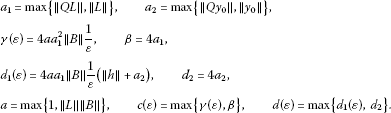Assume that $r\left(\epsilon \right)\ge d\left(\epsilon \right)+Cc\left(\epsilon \right)$. Then by (7) we have

$\begin{array}{rl}\parallel w\parallel & \le \parallel QLB\parallel \parallel {\left(\epsilon I+\mathrm{\Gamma }J\right)}^{-1}\left(h-Q{y}_{0}-QLF\left(y,v\right)\right)\parallel \\ \le \frac{1}{\epsilon }\parallel QL\parallel \parallel B\parallel \left(\parallel h\parallel +\parallel Q{y}_{0}\parallel +C\parallel QL\parallel \right)\\ =\frac{{d}_{1}\left(\epsilon \right)}{4}+\frac{\gamma \left(\epsilon \right)}{4{a}_{1}}C\\ \le \frac{{d}_{1}\left(\epsilon \right)}{4a}+\frac{\gamma \left(\epsilon \right)}{4a}C\le \frac{1}{4a}\left(d\left(\epsilon \right)+Cc\left(\epsilon \right)\right),\end{array}$

and

$\begin{array}{rl}\parallel z\parallel & \le \parallel {y}_{0}\parallel +\parallel LB\parallel \parallel w\parallel +\parallel L\parallel \parallel F\left(y,v\right)\parallel \\ \le {a}_{2}+\parallel L\parallel \parallel B\parallel \frac{1}{4a}\left(d\left(\epsilon \right)+Cc\left(\epsilon \right)\right)+\parallel L\parallel C\\ \le \frac{d\left(\epsilon \right)}{4}+\frac{1}{4}\left(d\left(\epsilon \right)+c\left(\epsilon \right)C\right)+\frac{c\left(\epsilon \right)}{4}C\\ \le \frac{1}{2}\left(d\left(\epsilon \right)+c\left(\epsilon \right)C\right).\end{array}$

Thus we proved that ${T}^{\epsilon }$ maps ${B}_{\epsilon }=\left\{\left(z,w\right)\in Y×V:\parallel \left(z,w\right)\parallel \le r\left(\epsilon \right)\right\}$ into itself. On the other hand, the operator ${T}^{\epsilon }$ is continuous and ${T}^{\epsilon }\left({B}_{\epsilon }\right)$ is relatively compact. By Schauder’s fixed-point theorem, for all $\epsilon >0$, ${T}^{\epsilon }$ has a fixed point in the ball ${B}_{\epsilon }$.

Step 2. Assume $\overline{QR\left(L,0\right)}=X$. By Step 1, the operator (4) has a fixed point $\left({y}_{\ast }^{\epsilon },{v}_{\ast }^{\epsilon }\right)$. So, $\left({y}_{\ast }^{\epsilon },{v}_{\ast }^{\epsilon }\right)$ satisfies (5) and, moreover, it follows that for all $h\in X$

$Q{y}_{\ast }^{\epsilon }-h=-\epsilon {\left(\epsilon I+\mathrm{\Gamma }J\right)}^{-1}\left(h-Q{y}_{0}-QLF\left({y}_{\ast }^{\epsilon },{v}_{\ast }^{\epsilon }\right)\right).$
(8)

So, ${z}_{\epsilon }:=Q{y}_{\ast }^{\epsilon }-h$ is a solution of the equation

$\epsilon {z}_{\epsilon }+\mathrm{\Gamma }J\left({z}_{\epsilon }\right)=\epsilon \left(QLF\left({y}_{\ast }^{\epsilon },{v}_{\ast }^{\epsilon }\right)+Q{y}_{0}-h\right).$
(9)

By the assumptions (A1) and (A2), the operator F is continuous bounded and L is compact. So, there exists a subsequence, still denoted by $\left\{F\left({y}_{\ast }^{\epsilon },{v}_{\ast }^{\epsilon }\right)\right\}$, which weakly converges to say $z\in Y$ and $LF\left({y}_{\ast }^{\epsilon },{v}_{\ast }^{\epsilon }\right)\to Lz$ strongly in Y as $\epsilon \to {0}^{+}$. From (7) and strong convergence of the sequence $\left\{h\left({z}_{\epsilon }\right)=h-Q{y}_{0}-QLF\left({y}_{\ast }^{\epsilon },{v}_{\ast }^{\epsilon }\right)\right\}$, it is easy to see that there exists ${C}_{1}>0$ such that for all $\epsilon >0$

$\parallel {z}_{\epsilon }\parallel =\parallel J\left({z}_{\epsilon }\right)\parallel \le \parallel QLF\left({y}_{\ast }^{\epsilon },{v}_{\ast }^{\epsilon }\right)+Q{y}_{0}-h\parallel \le {C}_{1}.$

Then we can extract a subsequence, still denoted by ${z}_{\epsilon }$, such that

for some ${\overline{z}}_{0}\in Z$. Applying $J\left({\overline{z}}_{0}\right)$ to equation (9) and taking the limit, we obtain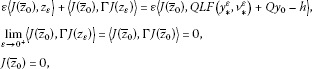since Γ is positive. So, $J\left({z}_{\epsilon }\right)⇀0$ as $\epsilon \to {0}^{+}$. Now, applying $J\left({z}_{\epsilon }\right)$ to equation (9), dividing through by ε and taking the limit, we obtain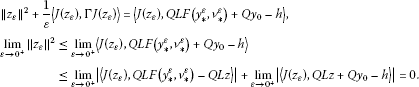Thus ${lim}_{\epsilon \to {0}^{+}}\parallel Q{y}_{\ast }^{\epsilon }-h\parallel =0$, consequently $\overline{QR\left(L,F\right)}=X$. The theorem is proved. □

## 3 Fractional integrodifferential equations

The purpose of this section is to establish sufficient conditions for the approximate controllability of certain classes of abstract fractional integrodifferential equations of the form

$\left\{\begin{array}{c}{}^{c}D_{t}^{\alpha }x\left(t\right)=Ax\left(t\right)+Bu\left(t\right)+f\left(t,x\left(t\right),{\int }_{0}^{t}g\left(t,s,x\left(s\right)\right)\phantom{\rule{0.2em}{0ex}}ds\right),\phantom{\rule{1em}{0ex}}t\in \left[0,b\right],\hfill \\ x\left(0\right)={x}_{0},\hfill \end{array}$
(10)

where the state variable x takes values in a separable reflexive Banach space X; ${}^{c}D^{\alpha }$ is the Caputo fractional derivative of order $\frac{1}{2}<\alpha <1$; A is the infinitesimal generator of a ${C}_{0}$ semigroup $S\left(t\right)$ of bounded operators on X; the control function u is given in ${L}_{2}\left(\left[0,b\right],U\right)$, U is a Hilbert space; B is a bounded linear operator from U into X, $\mathrm{\Delta }=\left\{\left(t,s\right):0\le s\le t\le T\right\}$ and $g:\mathrm{\Delta }×X\to X$, $f:I×X×X\to X$ are continuous bounded functions and ${x}_{0}\in X$.

Definition 5 The fractional integral of order α with the lower limit 0 for a function f is defined as

${I}^{\alpha }f\left(t\right)=\frac{1}{\gamma \left(\alpha \right)}{\int }_{0}^{t}\frac{f\left(s\right)}{{\left(t-s\right)}^{1-\alpha }}\phantom{\rule{0.2em}{0ex}}ds,\phantom{\rule{1em}{0ex}}t>0,\alpha >0,$

provided the right-hand side is pointwise defined on $\left[0,\mathrm{\infty }\right)$, where γ is the gamma function.

Definition 6 Riemann-Liouville derivative of order α with the lower limit 0 for a function $f:\left[0,\mathrm{\infty }\right)\to R$ can be written as

${}^{L}D^{\alpha }f\left(t\right)=\frac{1}{\gamma \left(n-\alpha \right)}\frac{{d}^{n}}{d{t}^{n}}{\int }_{0}^{t}\frac{f\left(s\right)}{{\left(t-s\right)}^{\alpha +1}}\phantom{\rule{0.2em}{0ex}}ds,\phantom{\rule{1em}{0ex}}t>0,n-1<\alpha

Definition 7 The Caputo derivative of order α for a function $f:\left[0,\mathrm{\infty }\right)\to R$ can be written as

${}^{c}D^{\alpha }f\left(t\right){=}^{L}{D}^{\alpha }\left(f\left(t\right)-\sum _{k=0}^{n-1}\frac{{t}^{k}}{k!}{f}^{\left(k\right)}\left(0\right)\right),\phantom{\rule{1em}{0ex}}t>0,n-1<\alpha

Remark 8

1. (1)

If $f\left(t\right)\in {C}^{n}\left[0,\mathrm{\infty }\right)$, then

${}^{c}D^{\alpha }f\left(t\right)=\frac{1}{\gamma \left(n-\alpha \right)}{\int }_{0}^{t}\frac{{f}^{\left(n\right)}\left(s\right)}{{\left(t-s\right)}^{\alpha +1-n}}\phantom{\rule{0.2em}{0ex}}ds={I}^{n-\alpha }{f}^{\left(n\right)}\left(t\right),\phantom{\rule{1em}{0ex}}t>0,n-1<\alpha
2. (2)

The Caputo derivative of a constant is equal to zero.

3. (3)

If f is an abstract function with values in X, then the integrals which appear in the above definitions are taken in Bochner’s sense.

For basic facts about fractional integrals and fractional derivatives, one can refer to .

In order to define the concept of a mild solution for problem (10), we associate problem (10) to the integral equation

$\begin{array}{rcl}x\left(t\right)& =& {\stackrel{ˆ}{S}}_{\alpha }\left(t\right){x}_{0}+{\int }_{0}^{t}{\left(t-s\right)}^{q-1}{S}_{\alpha }\left(t-s\right)f\left(s,x\left(s\right),{\int }_{0}^{s}g\left(s,r,x\left(r\right)\right)\phantom{\rule{0.2em}{0ex}}dr\right)\phantom{\rule{0.2em}{0ex}}ds\\ +{\int }_{0}^{t}{\left(t-s\right)}^{q-1}{S}_{\alpha }\left(t-s\right)Bu\left(s\right)\phantom{\rule{0.2em}{0ex}}ds,\end{array}$
(11)

whereand ${\eta }_{\alpha }$ is a probability density function defined on $\left(0,\mathrm{\infty }\right)$, that is, ${\eta }_{\alpha }\left(\theta \right)\ge 0$, $\theta \in \left(0,\mathrm{\infty }\right)$ and ${\int }_{0}^{\mathrm{\infty }}{\eta }_{\alpha }\left(\theta \right)\phantom{\rule{0.2em}{0ex}}d\theta =1$.

Lemma 9 

For any fixed $t\ge 0$, the operators ${\stackrel{ˆ}{S}}_{\alpha }\left(t\right)$ and ${S}_{\alpha }\left(t\right)$ are linear compact and bounded operators, i.e., for any $x\in X$, $\parallel {\stackrel{ˆ}{S}}_{\alpha }\left(t\right)x\parallel \le M\parallel x\parallel$ and $\parallel {S}_{\alpha }\left(t\right)x\parallel \le \frac{M}{\mathrm{\Gamma }\left(\alpha \right)}\parallel x\parallel$.

Definition 10 A solution $x\left(\cdot ;{x}_{0},u\right)\in C\left(\left[0,b\right],X\right)$ is said to be a mild solution of (10) if for any $u\in {L}_{2}\left(\left[0,b\right],U\right)$ and the integral equation (11) is satisfied.

Let ${x}_{b}\left({x}_{0};u\right)$ be the state value of (10) at terminal time b corresponding to the control u and the initial value ${x}_{0}$. Introduce the set $\mathrm{\Re }\left(b,{x}_{0}\right)=\left\{{x}_{b}\left({x}_{0};u\right)\left(0\right):u\in {L}_{2}\left(\left[0,b\right],U\right)\right\}$, which is called the reachable set of system (10) at terminal time b, its closure in X is denoted by $\overline{\mathrm{\Re }\left(b,{x}_{0}\right)}=X$.

Definition 11 System (10) is said to be approximately controllable on J if $\overline{\mathrm{\Re }\left(b,{x}_{0}\right)}=X$, that is, given an arbitrary $ϵ>0$, it is possible to steer from the point ${x}_{0}$ to within a distance ϵ from all points in the state space X at time b.

Consider the following linear fractional differential system:

$\begin{array}{r}{D}_{t}^{\alpha }x\left(t\right)=Ax\left(t\right)+Bu\left(t\right),\phantom{\rule{1em}{0ex}}t\in \left[0,b\right],\\ x\left(0\right)={x}_{0}.\end{array}$
(12)

The approximate controllability for linear fractional system (12) is a natural generalization of the approximate controllability of a linear first-order control system. It is convenient at this point to introduce the controllability operator associated with (12) as

${\mathrm{\Gamma }}_{0}^{b}={\int }_{0}^{b}{\left(b-s\right)}^{2\left(\alpha -1\right)}{S}_{\alpha }\left(b-s\right)B{B}^{\ast }{S}_{\alpha }^{\ast }\left(b-s\right)\phantom{\rule{0.2em}{0ex}}ds:X\to X,$

where ${B}^{\ast }$ denotes the adjoint of B and ${S}_{\alpha }^{\ast }$ is the adjoint of ${S}_{\alpha }$. It is straightforward that the operator ${\mathrm{\Gamma }}_{0}^{b}$ is a linear bounded operator. By Theorem 2, linear fractional control system (12) is approximately controllable on $\left[0,b\right]$ if and only if for any $h\in X$, ${z}_{\epsilon }\left(h\right)=\epsilon {\left(\epsilon I+{\mathrm{\Gamma }}_{0}^{b}J\right)}^{-1}\left(h\right)$ converges strongly to zero as $\epsilon \to {0}^{+}$.

Proposition 12 If $S\left(t\right)$, $t>0$, are compact operators and $0<\frac{1}{p}<\alpha \le 1$, then the operator

${L}_{\alpha }f\left(t\right)={\int }_{0}^{t}{\left(t-s\right)}^{\alpha -1}{S}_{\alpha }\left(t-s\right)f\left(s\right)\phantom{\rule{0.2em}{0ex}}ds,\phantom{\rule{1em}{0ex}}f\in {L}^{p}\left(\left[0,b\right],X\right),t\in \left[0,b\right],$

is compact from ${L}^{p}\left(\left[0,b\right],X\right)$ into $C\left(\left[0,b\right],X\right)$.

Proof According to the infinite dimensional version of the Ascoli-Arzela theorem, we need to show that

1. (i)

for arbitrary $t\in \left[0,b\right]$, the set $\left\{{L}_{\alpha }f\left(t\right):{\parallel f\parallel }_{{L}^{p}}\le 1\right\}$ is relatively compact in $C\left(\left[0,b\right],X\right)$;

2. (ii)

for arbitrary $\eta >0$, there exists $\delta >0$ such that

To prove (i), fix $0 and define for $0<\eta and $\delta >0$ operators ${L}_{\alpha }^{\eta ,\delta }$ from ${L}^{p}\left(\left[0,b\right],X\right)$ into X

$\begin{array}{rcl}\left({L}_{\alpha }^{\eta ,\delta }f\right)\left(t\right)& =& \alpha {\int }_{0}^{t-\lambda }{\int }_{\delta }^{\mathrm{\infty }}\theta {\left(t-s\right)}^{\alpha -1}{\eta }_{\alpha }\left(\theta \right)S\left({\left(t-s\right)}^{\alpha }\theta \right)f\left(s\right)\phantom{\rule{0.2em}{0ex}}ds\\ =& \alpha S\left({\lambda }^{\alpha }\delta \right){\int }_{0}^{t-\lambda }{\int }_{\delta }^{\mathrm{\infty }}\theta {\left(t-s\right)}^{\alpha -1}{\eta }_{\alpha }\left(\theta \right)S\left({\left(t-s\right)}^{\alpha }\theta -{\lambda }^{\alpha }\delta \right)f\left(s\right)\phantom{\rule{0.2em}{0ex}}ds,\\ f\in {L}^{p}\left(\left[0,b\right],X\right).\end{array}$

Since $S\left(t\right)$, $t>0$, is a compact operator, the operators ${L}_{\alpha }^{\eta ,\delta }$ are compact. Moreover, we have

$\begin{array}{rcl}\parallel \left({L}_{\alpha }f\right)\left(t\right)-\left({L}_{\alpha }^{\eta ,\delta }f\right)\left(t\right)\parallel & \le & \alpha \parallel {\int }_{0}^{t}{\int }_{0}^{\delta }\theta {\left(t-s\right)}^{\alpha -1}{\eta }_{\alpha }\left(\theta \right)S\left({\left(t-s\right)}^{\alpha }\theta \right)f\left(s\right)\phantom{\rule{0.2em}{0ex}}d\theta \phantom{\rule{0.2em}{0ex}}ds\parallel \\ +\alpha \parallel {\int }_{t-\lambda }^{t}{\int }_{\delta }^{\mathrm{\infty }}\theta {\left(t-s\right)}^{\alpha -1}{\eta }_{\alpha }\left(\theta \right)S\left({\left(t-s\right)}^{\alpha }\theta \right)f\left(s\right)\phantom{\rule{0.2em}{0ex}}d\theta \phantom{\rule{0.2em}{0ex}}ds\parallel \\ =:& {J}_{1}+{J}_{2}.\end{array}$

One can estimate ${J}_{1}$ and ${J}_{2}$ as follows:

$\begin{array}{rl}{J}_{1}& \le \alpha M{\int }_{0}^{t}{\left(t-s\right)}^{\alpha -1}\parallel f\left(s\right)\parallel \phantom{\rule{0.2em}{0ex}}ds\left({\int }_{0}^{\delta }\theta {\eta }_{\alpha }\left(\theta \right)\phantom{\rule{0.2em}{0ex}}d\theta \right)\\ \le \alpha M{\left({\int }_{0}^{t}{\left(t-s\right)}^{\left(\alpha -1\right)q}\phantom{\rule{0.2em}{0ex}}ds\right)}^{1/q}{\parallel f\parallel }_{{L}^{p}}\left({\int }_{0}^{\delta }\theta {\eta }_{\alpha }\left(\theta \right)\phantom{\rule{0.2em}{0ex}}d\theta \right),\end{array}$

and

$\begin{array}{rl}{J}_{2}& \le \alpha M{\int }_{t-\lambda }^{t}{\left(t-s\right)}^{\alpha -1}\parallel f\left(s\right)\parallel \phantom{\rule{0.2em}{0ex}}ds\left({\int }_{\delta }^{\mathrm{\infty }}\theta {\eta }_{\alpha }\left(\theta \right)\phantom{\rule{0.2em}{0ex}}d\theta \right)\\ \le \frac{\alpha M}{\gamma \left(1+\alpha \right)}{\left({\int }_{0}^{t}{\left(t-s\right)}^{\left(\alpha -1\right)q}\phantom{\rule{0.2em}{0ex}}ds\right)}^{1/q}{\left({\int }_{0}^{t}{\parallel f\left(s\right)\parallel }^{p}\phantom{\rule{0.2em}{0ex}}ds\right)}^{1/p}\\ =\frac{\alpha M}{\gamma \left(1+\alpha \right)}{\left(\frac{{\eta }^{\left(\alpha -1\right)q+1}}{\left(\alpha -1\right)q+1}\right)}^{1/q}{\parallel f\parallel }_{{L}^{p}},\end{array}$

where we have used the equality

${\int }_{0}^{\mathrm{\infty }}{\theta }^{\beta }{\eta }_{\alpha }\left(\theta \right)\phantom{\rule{0.2em}{0ex}}d\theta =\frac{\gamma \left(1+\beta \right)}{\gamma \left(1+\alpha \beta \right)}.$

Consequently, ${L}_{\alpha }^{\eta ,\delta }\to {L}_{\alpha }$ in the operator norm so that ${L}_{\alpha }$ is compact and (i) follows immediately.

To prove (ii), note that, for $0\le t\le t+h\le b$ and ${\parallel f\parallel }_{{L}^{p}}\le 1$, we haveApplying the Hölder inequality, we obtain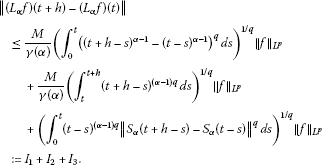It is clear that ${I}_{1},{I}_{2}\to 0$ as $h\to 0$. On the other hand, the compactness of $S\left(t\right)$, $t>0$ (and consequently ${S}_{\alpha }\left(t\right)$), implies the continuity of ${S}_{\alpha }\left(t\right)$, $t>0$, in the uniform operator topology. Then, by the Lebesque dominated convergence theorem, ${I}_{3}\to 0$ as $h\to 0$. Thus the proof of (ii), and therefore the proof of the proposition, is complete. □

Theorem 13 Suppose $S\left(t\right)$, $t>0$, is compact and $\frac{1}{2}<\alpha \le 1$. Then system (10) is approximately controllable on $\left[0,b\right]$ if the corresponding linear system is approximately controllable on $\left[0,b\right]$.

Proof Let $Y={L}_{2}\left(\left[0,b\right],X\right)$, $V={L}_{2}\left(\left[0,b\right],U\right)$, and ${y}_{0}={S}_{\alpha }\left(\cdot \right){x}_{0}\in Y$. Define the linear operators Q, L, ${L}_{1}$ and the nonlinear operator F by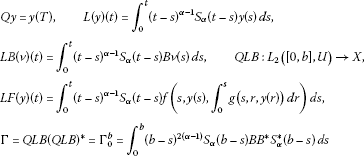for $y\in Y$, $v\in V$. It is easy to see that by Proposition 12 all the conditions of Theorem 4 are satisfied and (10) is approximately controllable. This completes the proof. □

## 4 Application

Consider the partial differential system of the form

$\left\{\begin{array}{c}{D}_{t}^{\alpha }x\left(t,\theta \right)={x}_{\theta \theta }\left(t,\theta \right)+b\left(\theta \right)u\left(t\right)+f\left(t,x\left(t,\theta \right),{\int }_{0}^{t}g\left(t,s,x\left(s,\theta \right)\right)\phantom{\rule{0.2em}{0ex}}ds\right),\hfill \\ x\left(t,0\right)=x\left(t,\pi \right)=0,\phantom{\rule{1em}{0ex}}t>0,\hfill \\ x\left(0\right)={x}_{0},\phantom{\rule{1em}{0ex}}0<\theta <\pi ,0\le t\le b,\hfill \end{array}$
(13)

where $u\in {L}_{2}\left[0,b\right]$, $X={L}_{2}\left[0,\pi \right]$, $h\in X$, $\frac{1}{2}<\alpha <1$, and $f:R×R\to R$, $g:R×R×R\to R$ are continuous and uniformly bounded. Let $B\in L\left(R,X\right)$ be defined as

$\left(Bu\right)\left(\theta \right)=b\left(\theta \right)u,\phantom{\rule{2em}{0ex}}{B}^{\ast }h={\int }_{0}^{\pi }h\left(\theta \right)b\left(\theta \right)\phantom{\rule{0.2em}{0ex}}d\theta ,$

where $0\le \theta \le \pi$, $u\in R$, $b\left(\theta \right)\in {L}_{2}\left[0,\pi \right]$, and let $A:X\to X$ be an operator defined by $Az={z}^{\mathrm{\prime }\mathrm{\prime }}$ with the domain

Then

$Az=\sum _{n=1}^{\mathrm{\infty }}\left(-{n}^{2}\right)\left(z,{e}_{n}\right){e}_{n},\phantom{\rule{1em}{0ex}}z\in D\left(A\right),$

where ${e}_{n}\left(\theta \right)=\sqrt{2/\pi }sinn\theta$, $0\le x\le \pi$, $n=1,2,\dots$ . It is known that A generates a compact semigroup $S\left(t\right)$, $t>0$, in X and is given by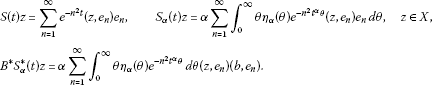Then ${B}^{\ast }{S}_{\alpha }^{\ast }\left(t\right)z=0$ for $0\le t implies

Now if $\left(b,{e}_{n}\right)\ne 0$ for all n, then $\left(z,{e}_{n}\right)=0$ for all n and $z=0$. Therefore, the associated linear system is approximately controllable provided that ${\int }_{0}^{\pi }b\left(\theta \right){e}_{n}\left(\theta \right)\phantom{\rule{0.2em}{0ex}}d\theta \ne 0$ for $n=1,2,3,\dots$ . Because of the compactness of the semigroup $S\left(t\right)$ (and consequently ${\stackrel{ˆ}{S}}_{\alpha }\left(t\right)$, ${S}_{\alpha }\left(t\right)$) generated by A, the associated linear system of (13) is not completely controllable but it is approximately controllable. Hence, according to Theorem 13, system (13) will be approximately controllable on $\left[0,b\right]$.

## 5 Conclusion

In this paper, abstract results concerning the approximate controllability of semilinear evolution systems in a separable reflexive Banach space are obtained. An approximate controllability result for semilinear systems is obtained by means of Schauder’s fixed-point theorem under the compactness assumption. It is also proven that the controllability of the linear system implies the controllability of the associated semilinear system. Then the obtained results are applied to derive sufficient conditions for the approximate controllability of the semilinear fractional integrodifferential equations in Banach spaces. Upon making some appropriate assumptions, by employing the ideas and techniques as in this paper, one can establish the approximate controllability results for a wide class of fractional deterministic and stochastic differential equations.

## References

1. Wang J, Zhou Y: Existence and controllability results for fractional semilinear differential inclusions. Nonlinear Anal., Real World Appl. 2011, 12: 3642-3653. 10.1016/j.nonrwa.2011.06.021

2. Tai Z: Controllability of fractional impulsive neutral integrodifferential systems with a nonlocal Cauchy condition in Banach spaces. Appl. Math. Lett. 2011, 24(12):2158-2161. 10.1016/j.aml.2011.06.018

3. Wang JR, Zhou Y: Complete controllability of fractional evolution systems. Commun. Nonlinear Sci. Numer. Simul. 2012, 17(11):4346-4355. 10.1016/j.cnsns.2012.02.029

4. Wang JR, Fan Z, Zhou Y: Nonlocal controllability of semilinear dynamic systems with fractional derivative in Banach spaces. J. Optim. Theory Appl. 2012, 154(1):292-302. 10.1007/s10957-012-9999-3

5. Wang J, Zhou Y, Wei W: A class of fractional delay nonlinear integrodifferential controlled systems in Banach spaces. Commun. Nonlinear Sci. Numer. Simul. 2011, 16(10):4049-4059. 10.1016/j.cnsns.2011.02.003

6. Zhou H: Approximate controllability for a class of semilinear abstract equations. SIAM J. Control Optim. 1983, 21: 551-555. 10.1137/0321033

7. Naito K: Approximate controllability for trajectories of semilinear control systems. J. Optim. Theory Appl. 1989, 60: 57-65. 10.1007/BF00938799

8. Li X, Yong J: Optimal Control Theory for Infinite Dimensional Systems. Birkhäuser, Boston; 1995.

9. Bian WM: Constrained controllability of some nonlinear systems. Appl. Anal. 1999, 72: 57-73. 10.1080/00036819908840730

10. Carmichel N, Quinn MD Lecture Notes in Control and Information Sciences 75. In Fixed Point Methods in Nonlinear Control. Springer, Berlin; 1984:24-51.

11. Zhang X: Exact controllability of semilinear evolution systems and its application. J. Optim. Theory Appl. 2000, 107: 415-432. 10.1023/A:1026460831701

12. Seidmann TI: Invariance of the reachable set under nonlinear perturbations. SIAM J. Control Optim. 1985, 25: 1173-1191.

13. Laziecka I, Triggiani R: Exact controllability of semilinear abstract systems with applications to waves and plates boundary control problems. Appl. Math. Optim. 1991, 23: 109-154. 10.1007/BF01442394

14. Balachandran K, Sakthivel R: Controllability of functional semilinear integrodifferential systems in Banach spaces. J. Math. Anal. Appl. 2001, 255(2):447-457. 10.1006/jmaa.2000.7234

15. Balachandran K, Anandhi ER: Controllability of neutral functional integrodifferential infinite delay systems in Banach spaces. Nonlinear Anal. 2005, 61: 405-423. 10.1016/j.na.2004.12.002

16. Balachandran K, Sakthivel R: Controllability of integrodifferential systems in Banach spaces. Appl. Math. Comput. 2001, 118: 63-71. 10.1016/S0096-3003(00)00040-0

17. Bashirov AE, Mahmudov NI: On concepts of controllability for deterministic and stochastic systems. SIAM J. Control Optim. 1999, 37(6):1808-1821. 10.1137/S036301299732184X

18. Chang Y-K, Chalishajar DN: Controllability of mixed Volterra-Fredholm-type integro-differential inclusions in Banach spaces. J. Franklin Inst. 2008, 345(5):499-507. 10.1016/j.jfranklin.2008.02.002

19. Fu X: Controllability of neutral functional differential systems in abstract space. Appl. Math. Comput. 2003, 141(2-3):281-296. 10.1016/S0096-3003(02)00253-9

20. Hernandez M E, O’Regan D: Controllability of Volterra-Fredholm type systems in Banach spaces. J. Franklin Inst. 2009, 346(2):95-101. 10.1016/j.jfranklin.2008.08.001

21. Ji S, Li G, Wang M: Controllability of impulsive differential systems with nonlocal conditions. Appl. Math. Comput. 2011, 217(16):6981-6989. 10.1016/j.amc.2011.01.107

22. Klamka J: Schauder’s fixed-point theorem in nonlinear controllability problems. Control Cybern. 2000, 29(1):153-165.

23. Klamka J: Constrained approximate controllability. IEEE Trans. Autom. Control 2000, 45(9):1745-1749. 10.1109/9.880640

24. Klamka J: Constrained controllability of semilinear delayed systems. Bull. Pol. Acad. Sci., Tech. Sci. 2001, 49(3):505-515.

25. Klamka J: Constrained controllability of semilinear systems. Nonlinear Anal. 2001, 47: 2939-2949. 10.1016/S0362-546X(01)00415-1

26. Klamka J: Constrained exact controllability of semilinear systems. Syst. Control Lett. 2002, 4(2):139-147.

27. Dauer JP, Mahmudov NI: Controllability of some nonlinear systems in Hilbert spaces. J. Optim. Theory Appl. 2004, 123(2):319-329. 10.1007/s10957-004-5151-3

28. Dauer JP, Mahmudov NI: Approximate controllability of semilinear functional equations in Hilbert spaces. J. Math. Anal. Appl. 2002, 273(2):310-327. 10.1016/S0022-247X(02)00225-1

29. Debbouche A, Baleanu D: Controllability of fractional evolution nonlocal impulsive quasilinear delay integro-differential systems. Comput. Math. Appl. 2011, 62: 1442-1450. 10.1016/j.camwa.2011.03.075

30. Mahmudov NI: On controllability of linear stochastic systems. IEEE Trans. Autom. Control 2001, 46: 724-732. 10.1109/9.920790

31. Mahmudov NI: Approximate controllability of semilinear deterministic and stochastic evolution equations in abstract spaces. SIAM J. Control Optim. 2003, 42: 1604-1622. 10.1137/S0363012901391688

32. Mahmudov NI: Approximate controllability of evolution systems with nonlocal conditions. Nonlinear Anal., Theory Methods Appl. 2008, 68: 536-546. 10.1016/j.na.2006.11.018

33. Curtain RF, Zwart HJ: An Introduction to Infinite Dimensional Linear Systems Theory. Springer, New York; 1995.

34. Zhou Y, Jiao F: Nonlocal Cauchy problem for fractional evolution equations. Nonlinear Anal., Real World Appl. 2010, 11: 4465-4475. 10.1016/j.nonrwa.2010.05.029

35. Abada N, Benchohra M, Hammouche H: Existence and controllability results for nondensely defined impulsive semilinear functional differential inclusions. J. Differ. Equ. 2009, 246: 3834-3863. 10.1016/j.jde.2009.03.004

36. Górniewicz L, Ntouyas SK, O’Regan D: Controllability of semilinear differential equations and inclusions via semigroup theory in Banach spaces. Rep. Math. Phys. 2005, 56: 437-470. 10.1016/S0034-4877(05)80096-5

37. Fu X: Controllability of non-densely defined functional differential systems in abstract space. Appl. Math. Lett. 2006, 19: 369-377. 10.1016/j.aml.2005.04.016

38. Sakthivel R, Ren Y, Mahmudov NI: Approximate controllability of second-order stochastic differential equations with impulsive effects. Mod. Phys. Lett. B 2010, 24: 1559-1572. 10.1142/S0217984910023359

39. Sakthivel R, Ren Y: Approximate controllability of fractional differential equations with state-dependent delay. Results Math. 2012. doi:10.1007/s00025-012-0245-y

40. Sakthivel R, Nieto JJ, Mahmudov NI: Approximate controllability of nonlinear deterministic and stochastic systems with unbounded delay. Taiwan. J. Math. 2010, 14: 1777-1797.

41. Sakthivel R, Mahmudov NI, Nieto JJ: Controllability for a class of fractional-order neutral evolution control systems. Appl. Math. Comput. 2012, 218(20):10334-10340. 10.1016/j.amc.2012.03.093

42. Rykaczewski K: Approximate controllability of differential inclusions in Hilbert spaces. Nonlinear Anal., Theory Methods Appl. 2012, 75(5):2701-2712. 10.1016/j.na.2011.10.049

43. Ren Y, Hu L, Sakthivel R: Controllability of impulsive neutral stochastic functional differential inclusions with infinite delay. J. Comput. Appl. Math. 2011, 235(8):2603-2614. 10.1016/j.cam.2010.10.051

44. Sakthivel R, Ren Y, Mahmudov NI: On the approximate controllability of semilinear fractional differential systems. Comput. Math. Appl. 2011, 62(3):1451-1459. 10.1016/j.camwa.2011.04.040

45. Sakthivel R, Suganya S, Anthoni SM: Approximate controllability of fractional stochastic evolution equations. Comput. Math. Appl. 2012, 63(3):660-668. 10.1016/j.camwa.2011.11.024

46. Sakthivel R, Ren Y: Complete controllability of stochastic evolution equations with jumps. Rep. Math. Phys. 2011, 68(2):163-174. 10.1016/S0034-4877(12)60003-2

47. Sukavanam N, Kumar S: Approximate controllability of fractional order semilinear delay systems. J. Optim. Theory Appl. 2011, 151: 373-384. doi:10.1007/s10957-011-9905-4 10.1007/s10957-011-9905-4

48. Barbu V, Precupanu T Mathematics and Its Applications (East European Series) 10. In Convexity and Optimization in Banach Spaces. 2nd edition. Reidel, Dordrecht; 1986.

49. Samko SG, Kilbas AA, Marichev OI: Fractional Integrals and Derivatives. Theory and Applications. Gordon & Breach, London; 1993.

## Acknowledgements

Dedicated to Professor Hari M Srivastava.

The author would like to thank the reviewers for their valuable comments and helpful suggestions that improved the note’s quality.

## Author information

Authors

### Corresponding author

Correspondence to Nazim I Mahmudov.

### Competing interests

The author declares that they have no competing interests.

## Rights and permissions

Reprints and Permissions

Mahmudov, N.I. Approximate controllability of some nonlinear systems in Banach spaces. Bound Value Probl 2013, 50 (2013). https://doi.org/10.1186/1687-2770-2013-50

• Accepted:

• Published:

• DOI: https://doi.org/10.1186/1687-2770-2013-50

### Keywords

• Approximate Controllability
• Compactness Assumption
• Fractional Dynamical System
• Associate Linear System
• Separable Reflexive Banach Space